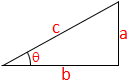# Sine Calculator

In mathematics, the sine is a trigonometric function of an angle. The sine of an acute angle is defined in the context of a right triangle: for the specified angle, it is the ratio of the length of the side that is opposite that angle to the length of the longest side of the triangle (the hypotenuse).sin = ?